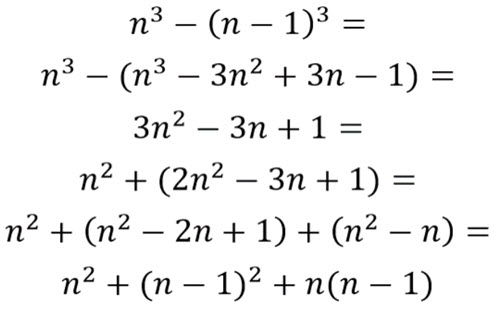Go to website

# Difference of cubes

The lesson aims to challenge students through exploring their understanding of square numbers through solving quadratic equations. Students work collaboratively in teams, exploring the difference between sequences. The lesson is aimed to challenge students' thinking, encouraging them to explore how difference in cube numbers can alter an answer.

Year level(s) Year 10
Audience Teacher
Purpose Extension, Student task, Teaching resource, Teaching strategies
Teaching strategies and pedagogical approaches Collaborative learning, Concrete Representational Abstract model, Differentiated teaching, Mathematics investigation, Setting goals, Structuring lessons, Worked examples
Keywords cube numbers, square roots, quadratic sequence, linear sequence

## Curriculum alignment

Curriculum connections Critical and creative thinking, Numeracy
Strand and focus Algebra, Apply understanding, Number, Build understanding
Topics Algebraic expressions, Addition and subtraction, Multiples, factors and powers, Multiplication and division, Operating with number
AC: Mathematics content descriptions
ACMNA233 Expand binomial products and factorise monic quadratic expressions using a variety of strategies
National numeracy learning progression Additive strategies
Multiplicative strategies
Number patterns and algebraic thinking - NPA9